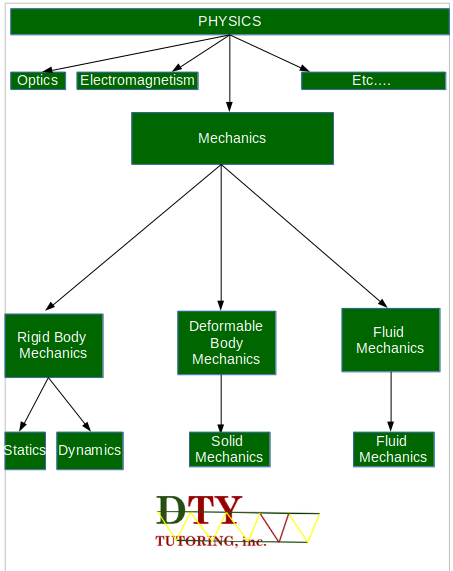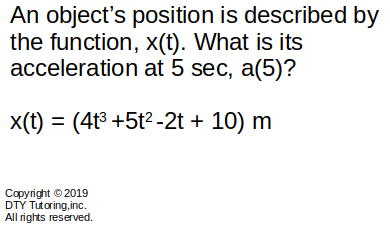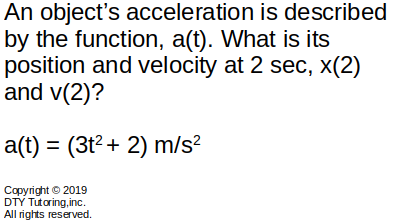/////////////////////////////////////////////////////////////\\\\\\\\\\\\\\\\\\\\\\\\\\\\\\\\\\\\\\\\\\\\\\\\Dedication Time consistencY

 σy↑⇀τyx

σx←τxy

# Physics-Classical Mechanics

Mechanics is a branch of physics that deals with motion and the behavior of bodies under the effect of forces.### Newton's Law of motion

1. First Law

"A body at rest will remain at rest, and a body in motion will remain in motion unless it is acted upon by an external force."(Equilibrium/Inertia)

2. Second Law

"The force acting on an object is equal to the mass of that object times its acceleration." (Accelerated motion, F = ma)

3. Third Law

"For every action, there is an equal and opposite reaction." (Action-reaction)

### Problems

1. Finding Normal force example 1
2. A box is sitting on the floor as shown on the figure below, calculate the normal force, N(in kN).PhysicsEx1solution.pdf

3. Finding the acceleration of an object at a certain time given position function
4. Find the acceleration of the object at t = 5 sec as described on the figure below.PhysicsEx2solution.pdf

5. Finding the position and velocity of an object at a certain time given acceleration function
6. Find
(a)the position of the object at t = 2 sec and,
(b)the velocity of the object at t = 2 sec
as described on the figure below.PhysicsEx3solution.pdf

### Videos

↾τxy→σx

 ↽τyx↓σy

 w(x)↧↧↧↧↧↧↧↧↧↧↧↧↧↧↧↧↧↧↧↧↧↧↧↧↧↧↧↧↧↧↧↧↧↧↧↧↧↧↧↧↧

 INQUIRE ABOUT FAQs Our contacts Our courses INFORMATION Our areas of interest Site Map About our company FOLLOW US ON